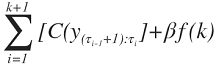# 1.1 - 8.10 - Change-Point Detection Functions (ML Engine) - Teradata Vantage

## Teradata Vantage™ - Machine Learning Engine Analytic Function Reference

Product
Release Number
1.1
8.10
Published
October 2019
Content Type
Programming Reference
Publication ID
B700-4003-079K
Language
English (United States)

Change-point detection functions detect the change points in a stochastic process or time series. These functions take sorted time series data as input and output change points or data segments.

In statistical analysis, change detection or change-point detection tries to identify the abrupt changes of a stochastic process or time series.

Consider the following ordered time series data sequence, where t is a time variable:

y(t), t=1, 2, ..., n

Change-point detection tries to find a segmented model M, given by the following equation:

Y = f 1(t, w 1) + e 1(t), (1 <t <=τ 1)

= f 2(t, w 2) + e 2(t), (τ 1 <t <=τ 2)

...

= f k (t, w k ) + e k (t), (τ k-1 <t <=τ k )

= f k+1(t, w k+1) + e k+1(t), (τ k <t <=n k )

where:

• f i(t,w 1) is the function (with its vector of parameters w i) that fits in segment i.
• Each τ i is the change point between successive segments.
• Each e i(t) is an error term.
• n is the size of data series and k is the number of change points.

Segmentation model selection aims to find the function f i(t,w 1) that best approximates the data of each segment. Various model selection methods have been proposed. According to literature, the most commonly used model selection method is normal distribution.

Search method selection aims to find the change points from a global perspective.

If τ0 =0 and τ k+1 =n, one common method of identifying the change point is to minimize this value:C is a cost function for a segment to measure the difference between f i(t,w 1) and the original data. βf (k) is a penalty to guard against over-fitting. The choice is linear in the number of change points k; that is, βf (k)k. There are information criteria for the evaluation, such as Akaike Information Criterion (AIC) and Bayes Information Criterion (BIC).

For AIC, β=2p, where p is the number of additional parameters introduced by adding a change point.

For BIC (also called SBIC), β=plog(n).

Function Description
ChangePointDetection (ML Engine) For when input data can be stored in memory.
ChangePointDetectionRT (ML Engine) For when input data cannot be stored in memory or application needs real-time response.